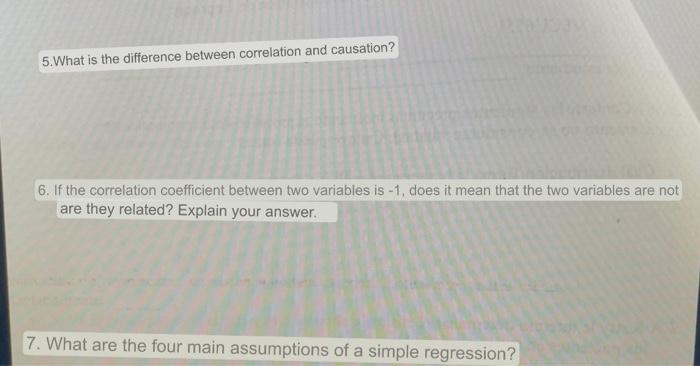# Question 5. What is the difference between correlation and causation? 6. If the correlation coefficient between two variables is -1, does it mean that the two variables are not are they related? Explain your answer. 7. What are the four main assumptions of a simple regression? 5. What is the difference between correlation and causation? 6. If the correlation coefficient between two variables is -1, does it mean that the two variables are not are they related? Explain your answer. 7. What are the four main assumptions of a simple regression?EQRLG2 The Asker · Probability and StatisticsTranscribed Image Text: 5. What is the difference between correlation and causation? 6. If the correlation coefficient between two variables is -1, does it mean that the two variables are not are they related? Explain your answer. 7. What are the four main assumptions of a simple regression?
More
Transcribed Image Text: 5. What is the difference between correlation and causation? 6. If the correlation coefficient between two variables is -1, does it mean that the two variables are not are they related? Explain your answer. 7. What are the four main assumptions of a simple regression?